$$\require{cancel}$$

11.6: Maxwell's Equations

•• Contributed by Benjamin Crowell
• Professor (Physics) at Fullerton College

11.6.1 Induced magnetic fieldsa / James Clerk Maxwell (1831-1879)

We are almost, but not quite, done figuring out the complete set of physical laws, called Maxwell's equations, governing electricity and magnetism. We are only missing one more term. For clarity, I'll state Maxwell's equations with the missing part included, and then discuss the physical motivation and experimental evidence for sticking it in:

Maxwell's equations

For any closed surface, the fluxes through the surface are

\begin{align*} \Phi_E &= 4\pi kq_{in}\\ \text{and}\\ \Phi_B &= 0 .\end{align*}

For any surface that is not closed,the circulations around the edges of the surface are given by

\begin{align*}\Gamma_E &= -\frac{\partial\Phi_B}{\partial t} \\ \text{and} \\ c^2\Gamma_B &= \frac{\partial\Phi_E}{\partial t} + 4\pi k I_{through} . \end{align*}

The $$\Phi_E$$ equation is Gauss' law: charges make diverging electric fields. The corresponding equation for $$\Phi_B$$ tells us that magnetic “charges” (monopoles) don't exist, so magnetic fields never diverge. The third equation says that changing magnetic fields induce curly electric fields, whose curliness we can measure using the emf, $$\Gamma_E$$, around a closed loop. The final equation, for $$\Gamma_B$$, is the only one where anything new has been added. Without the new time derivative term, this equation would simply be Ampère's law. (I've chosen to move the $$c^2$$ over to the left because it simplifies the writing, and also because it more clearly demonstrates the analogous roles played by charges and currents in the $$\Phi_E$$ and $$\Gamma_B$$ equations.)

This new $$\partial\Phi_E/\partial t$$ term says that just as a changing magnetic field can induce a curly electric field, a changing electric field can induce a curly magnetic field. Why should this be so? The following examples show that Maxwell's equations would not make sense in general without it.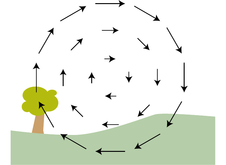b / Where is the moving charge responsible for this magnetic field?

Figure b shows a mysterious curly magnetic field. Magnetic fields are supposed to be made by moving charges, but there don't seem to be any moving charges in this landscape. Where are they? One reasonable guess would be that they're behind your head, where you can't see them. Suppose there's a positively charged particle about to hit you in the back of the head. This particle is like a current going into the page. We're used to dealing with currents made by many charged particles, but logically we can't have some minimum number that would qualify as a current. This is not a static current, however, because the current at a given point in space is not staying the same over time. If the particle is pointlike, then it takes zero time to pass any particular location, and the current is then infinite at that point in space. A moment later, when the particle is passing by some other location, there will be an infinite current there, and zero current in the previous location. If this single particle qualifies as a current, then it should be surrounded by a curly magnetic field, just like any other current.11

This explanation is simple and reasonable, but how do we know it's correct? Well, it makes another prediction, which is that the positively charged particle should be making an electric field as well. Not only that, but if it's headed for the back of your head, then it's getting closer and closer, so the electric field should be getting stronger over time. But this is exactly what Maxwell's equations require. There is no current $$I_{through}$$ piercing the Ampèrian surface shown in figure c, so Maxwell's equation for $$\Gamma_B$$ becomes $$c^2\Gamma_B = \partial\Phi_E/\partial t$$.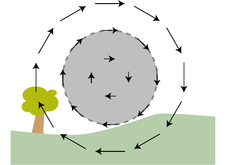c / An Ampèrian surface superimposed on the landscape.

The only reason for an electric field to change is if there are charged particles making it, and those charged particles are moving. When charged particles are moving, they make magnetic fields as well.

Note that the above example is also sufficient to prove the positive sign of the $$\partial\Phi_E/\partial t$$ term in Maxwell's equations, which is different from the negative sign of Faraday's $$-\partial\Phi_B/\partial t$$ term.

The addition of the $$\partial\Phi_E/\partial t$$ term has an even deeper and more important physical meaning. With the inclusion of this term, Maxwell's equations can describe correctly the way in which disturbances in the electric and magnetic fields ripple outwards at the speed of light. Indeed, Maxwell was the first human to understand that light was in fact an electromagnetic wave. Legend has it that it was on a starry night that he first realized this implication of his equations. He went for a walk with his wife, and told her she was the only other person in the world who really knew what starlight was.

To see how the $$\partial\Phi_E/\partial t$$ term relates to electromagnetic waves, let's look at an example where we would get nonsense without it.d / An electron jumps through a hoop.

Figure d shows an electron that sits just on one side of an imaginary Ampèrian surface, and then hops through it at some randomly chosen moment. Unadorned with the $$\partial\Phi_E/\partial t$$ term, Maxwell's equation for $$\Gamma_B$$ reads as $$c^2\Gamma_B = 4\pi k I_{through}$$, which is Ampère's law. If the electron is a pointlike particle, then we have an infinite current $$I_{through}$$ at the moment when it pierces the imaginary surface, and zero current at all other times. An infinite magnetic circulation $$\Gamma_B$$ can only be produced by an infinite magnetic field, so without the $$\partial\Phi_E/\partial t$$ term, Maxwell's equations predict nonsense: the edge of the surface would experience an infinite magnetic field at one instant, and zero magnetic field at all other times. Even if the infinity didn't upset us, it doesn't make sense that anything special would happen at the moment the electron passed through the surface, because the surface is an imaginary mathematical construct. We could just as well have chosen the curved surface shown in figure e, which the electron never crosses at all.e / An alternative Ampèrian surface.

We are already clearly getting nonsensical results by omitting the $$\partial\Phi_E/\partial t$$ term, and this shouldn't surprise us because Ampère's law only applies to statics. More to the point, Ampère's law doesn't have time in it, so it predicts that this effect is instantaneous. According to Ampère's law, we could send Morse code signals by wiggling the electron back and forth, and these signals would be received at distant locations instantly, without any time delay at all. This contradicts the theory of relativity, one of whose predictions is that information cannot be transmitted at speeds greater than the speed of light.

Discussion Questions

◊ Induced magnetic fields were introduced in the text via the imaginary landscape shown in figure b on page 695, and I argued that the magnetic field could have been produced by a positive charge coming from behind your head. This is a specific assumption about the number of charges (one), the direction of motion, and the sign of the charge. What are some other scenarios that could explain this field?

11.6.2 Light waves

We could indeed send signals using this scheme, and the signals would be a form of light. A radio transmitting antenna, for instance, is simply a device for whipping electrons back and forth at megahertz frequencies. Radio waves are just like visible light, but with a lower frequency. With the addition of the $$\partial\Phi_E/\partial t$$ term, Maxwell's equations are capable of describing electromagnetic waves. It would be possible to use Maxwell's equations to calculate the pattern of the electric and magnetic fields rippling outward from a single electron that fidgets at irregular intervals, but let's pick a simpler example to analyze.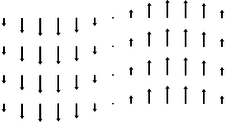f / A magnetic field in the form of a sine wave.

The simplest wave pattern is a sine wave like the one shown in figure f. Let's assume a magnetic field of this form, and see what Maxwell's equations tell us about it. If the wave is traveling through empty space, then there are no charges or currents present, and Maxwell's equations become

\begin{align*} \Phi_E &= 0 \\ \Phi_B &= 0 \\ \Gamma_E &= -\frac{\partial\Phi_B}{\partial t} \\ c^2\Gamma_B &= \frac{\partial\Phi_E}{\partial t} . \end{align*}g / The wave pattern is curly. For example, the circulation around this reactangle is nonzero and counterclockwise.

The equation $$\Phi=0$$ has already been verified for this type of wave pattern in example 36 on page 630. Even if you haven't learned the techniques from that section, it should be visually plausible that this field pattern doesn't diverge or converge on any particular point.

Geometry of the electric and magnetic fields

The equation $$c^2\Gamma_B=\partial\Phi_E/\partial t$$ tells us that there can be no such thing as a purely magnetic wave. The wave pattern clearly does have a nonvanishing circulation around the edge of the surface suggested in figure g, so there must be an electric flux through the surface. This magnetic field pattern must be intertwined with an electric field pattern that fills the same space. There is also no way that the two sides of the equation could stay synchronized with each other unless the electric field pattern is also a sine wave, and one that has the same wavelength, frequency, and velocity. Since the electric field is making a flux through the indicated surface, it's plausible that the electric field vectors lie in a plane perpendicular to that of the magnetic field vectors. The resulting geometry is shown in figure h. Further justification for this geometry is given later in this subsection.h / The geometry of an electromagnetic wave.

One feature of figure h that is easily justified is that the electric and magnetic fields are perpendicular not only to each other, but also to the direction of propagation of the wave. In other words, the vibration is sideways, like people in a stadium “doing the wave,” not lengthwise, like the accordion pattern in figure i. (In standard wave terminology, we say that the wave is transverse, not longitudinal.)i / An impossible wave pattern.

The wave pattern in figure i is impossible, because it diverges from the middle. For virtually any choice of Gaussian surface, the magnetic and electric fluxes would be nonzero, contradicting the equations $$\Phi_B=0$$ and $$\Phi_E=0$$.12
Example 23: Reflectionj / Example 23. The incident and reflected waves are drawn offset from each other for clarity, but are actually on top of each other so that their fields superpose.

The wave in figure j hits a silvered mirror. The metal is a good conductor, so it has constant voltage throughout, and the electric field equals zero inside it: the wave doesn't penetrate and is 100% reflected. If the electric field is to be zero at the surface as well, the reflected wave must have its electric field inverted (p. 362), so that the incident and reflected fields cancel there.

But the magnetic field of the reflected wave is not inverted. This is because the reflected wave has to have the correct right-handed relationship between the fields and the direction of propagation.

Polarization

Two electromagnetic waves traveling in the same direction through space can differ by having their electric and magnetic fields in different directions, a property of the wave called its polarization.

The speed of light

What is the velocity of the waves described by Maxwell's equations? Maxwell convinced himself that light was an electromagnetic wave partly because his equations predicted waves moving at the velocity of light, $$c$$. The only velocity that appears in the equations is $$c$$, so this is fairly plausible, although a real calculation is required in order to prove that the velocity of the waves isn't something like $$2c$$ or $$c/\pi$$ --- or zero, which is also $$c$$ multiplied by a constant! The following discussion, leading up to a proof that electromagnetic waves travel at $$c$$, is meant to be understandable even if you're reading this book out of order, and haven't yet learned much about waves. As always with proofs in this book, the reason to read it isn't to convince yourself that it's true, but rather to build your intuition. The style will be visual. In all the following figures, the wave patterns are moving across the page (let's say to the right), and it usually doesn't matter whether you imagine them as representing the wave's magnetic field or its electric field, because Maxwell's equations in a vacuum have the same form for both fields. Whichever field we imagine the figures as representing, the other field is coming in and out of the page.

The velocity of the waves is not zero. If the wave pattern was standing still in space, then the right sides of the $$\Gamma$$ equations would be zero, because there would be no change in the field over time at a particular point. But the left sides are not zero, so this is impossible.13

The velocity of the waves is a fixed number for a given wave pattern. Consider a typical sinusoidal wave of visible light, with a distance of half a micrometer from one peak to the next peak. Suppose this wave pattern provides a valid solution to Maxwell's equations when it is moving with a certain velocity. We then know, for instance, that there cannot be a valid solution to Maxwell's equations in which the same wave pattern moves with double that velocity. The time derivatives on the right sides of Maxwell's equations for $$\Gamma_E$$ and $$\Gamma_B$$ would be twice as big, since an observer at a certain point in space would see the wave pattern sweeping past at twice the rate. But the left sides would be the same, so the equations wouldn't equate.

The velocity is the same for all wave patterns. In other words, it isn't $$0.878c$$ for one wave pattern, and $$1.067c$$ for some other pattern. This is surprising, since, for example, water waves with different shapes do travel at different speeds. Similarly, even though we speak of “the speed of sound,” sound waves do travel at slightly different speeds depending on their pitch and loudness, although the differences are small unless you're talking about cannon blasts or extremely high frequency ultrasound. To see how Maxwell's equations give a consistent velocity, consider figure k.k / Red and blue light travel at the same speed.

Along the right and left edges of the same Ampèrian surface, the more compressed wave pattern of blue light has twice as strong a field, so the circulations on the left sides of Maxwell's equations are twice as large.14 To satisfy Maxwell's equations, the time derivatives of the fields must also be twice as large for the blue light. But this is true only if the blue light's wave pattern is moving to the right at the same speed as the red light's: if the blue light pattern is sweeping over an observer with a given velocity, then the time between peaks is half as much, like the clicking of the wheels on a train whose cars are half the length.15l / Bright and dim light travel at the same speed.

We can also check that bright and dim light, as shown in figure l, have the same velocity. If you haven't yet learned much about waves, then this might be surprising. A material object with more energy goes faster, but that's not the case for waves. The circulation around the edge of the Ampèrian surface shown in the figure is twice as strong for the light whose fields are doubled in strength, so the left sides of Maxwell's $$\Gamma$$ equations are doubled. The right sides are also doubled, because the derivative of twice a function is twice the derivative of the original function. Thus if dim light moving with a particular velocity is a solution, then so is bright light, provided that it has the same velocity.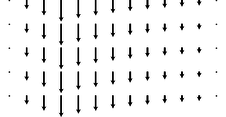m / A nonsinusoidal wave.

We can now see that all sinusoidal waves have the same velocity. What about nonsinusoidal waves like the one in figure m? There is a mathematical theorem, due to Fourier, that says any function can be made by adding together sinusoidal functions. For instance, $$3\sin x-7\cos 3x$$ can be made by adding together the functions $$3\sin x$$ and $$-7\cos 3x$$, but Fourier proved that this can be done even for functions, like figure m, that aren't obviously built out of sines and cosines in the first place. Therefore our proof that sinusoidal waves all have the same velocity is sufficient to demonstrate that other waves also have this same velocity.

We're now ready to prove that this universal speed for all electromagnetic waves is indeed $$c$$. Since we've already convinced ourselves that all such waves travel at the same speed, it's sufficient to find the velocity of one wave in particular. Let's pick the wave whose fields have magnitudes

\begin{align*} E &= \tilde{E}\sin(x+vt) \text{and}\\ B &= \tilde{B}\sin(x+vt) , \end{align*}

which is about as simple as we can get. The peak electric field of this wave has a strength $$\tilde{E}$$, and the peak magnetic field is $$\tilde{B}$$. The sine functions go through one complete cycle as $$x$$ increases by $$2\pi=6.28...$$, so the distance from one peak of this wave to the next --- its wavelength --- is 6.28... meters. This means that it is not a wave of visible light but rather a radio wave (its wavelength is on the same order of magnitude as the size of a radio antenna). That's OK. What was glorious about Maxwell's work was that it unified the whole electromagnetic spectrum. Light is simple. Radio waves aren't fundamentally any different than light waves, x-rays, or gamma rays. 16

The justification for putting $$x+vt$$ inside the sine functions is as follows. As the wave travels through space, the whole pattern just shifts over. The fields are zero at $$x=0$$, $$t=0$$, since the sine of zero is zero. This zero-point of the wave pattern shifts over as time goes by; at any time $$t$$ its location is given by $$x+vt=0$$. After one second, the zero-point is located at $$x=-(1\ \text{s})v$$. The distance it travels in one second is therefore numerically equal to $$v$$, and this is exactly the concept of velocity: how far something goes per unit time.

The wave has to satisfy Maxwell's equations for $$\Gamma_E$$ and $$\Gamma_B$$ regardless of what Ampèrian surfaces we pick, and by applying them to any surface, we could determine the speed of the wave. The surface shown in figure n turns out to result in an easy calculation: a narrow strip of width $$2\ell$$ and height $$h$$, coinciding with the position of the zero-point of the field at $$t=0$$.n / The magnetic field of the wave. The electric field, not shown, is perpendicular to the page.

Now let's apply the equation $$c^2\Gamma_B=\partial\Phi_E/\partial t$$ at $$t=0$$. Since the strip is narrow, we can approximate the magnetic field using $$\sin x\approx x$$, which is valid for small $$x$$. The magnetic field on the right edge of the strip, at $$x=\ell$$, is then $$\tilde{B}\ell$$, so the right edge of the strip contributes $$\tilde{B}\ell h$$ to the circulation. The left edge contributes the same amount, so the left side of Maxwell's equation is

$\begin{equation*} c^2\Gamma_B = c^2 \cdot 2 \tilde{B}\ell h . \end{equation*}$

The other side of the equation is

\begin{align*} \frac{\partial\Phi_E}{\partial t} = \frac{\partial}{\partial t}(EA) = 2\ell h \frac{\partial E}{\partial t} , \end{align*}
where we can dispense with the usual sum because the strip is narrow and there is no variation in the field as we go up and down the strip. The derivative equals $$v\tilde{E}\cos(x+vt)$$, and evaluating the cosine at $$x=0$$, $$t=0$$ gives
\begin{align*}\frac{\partial\Phi_E}{\partial t} = 2v \tilde{E}\ell h \\ \end{align*}

Maxwell's equation for $$\Gamma_B$$ therefore results in

\begin{align*} 2c^2\tilde{B}\ell h &= 2\tilde{E}\ell h v\\ c^2\tilde{B} &= v\tilde{E} . \end{align*}

An application of $$\Gamma_E=-\partial\Phi_B/\partial t$$ gives a similar result, except that there is no factor of $$c^2$$

$\begin{equation*} \tilde{E} = v \tilde{B} . \end{equation*}$

(The minus sign simply represents the right-handed relationship of the fields relative to their direction of propagation.)

Multiplying these last two equations by each other, we get

\begin{align*} c^2\tilde{B}\tilde{E} &= v^2\tilde{E}\tilde{B} \\ c^2 &= v^2 \\ v &= \pm c . \end{align*}

This is the desired result. (The plus or minus sign shows that the wave can travel in either direction.)

As a byproduct of this calculation, we can find the relationship between the strengths of the electric and magnetic fields in an electromagnetic wave. If, instead of multiplying the equations $$c^2\tilde{B} = v \tilde{E}$$ and $$\tilde{E} = v \tilde{B}$$, we divide them, we can easily show that $$\tilde{E}=c\tilde{B}$$.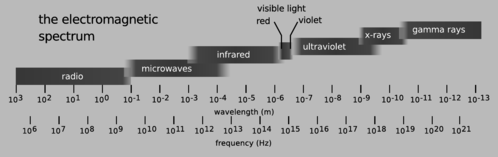o / The electromagnetic spectrum.

Figure o shows the complete spectrum of light waves. The wavelength $$\lambda$$ (number of meters per cycle) and frequency $$f$$ (number of cycles per second) are related by the equation $$c=f\lambda$$. Maxwell's equations predict that all light waves have the same structure, regardless of wavelength and frequency, so even though radio and x-rays, for example, hadn't been discovered, Maxwell predicted that such waves would have to exist. Maxwell's 1865 prediction passed an important test in 1888, when Heinrich Hertz published the results of experiments in which he showed that radio waves could be manipulated in the same ways as visible light waves. Hertz showed, for example, that radio waves could be reflected from a flat surface, and that the directions of the reflected and incoming waves were related in the same way as with light waves, forming equal angles with the surface. Likewise, light waves can be focused with a curved, dish-shaped mirror, and Hertz demonstrated the same thing with a dish-shaped radio antenna.

Momentum of light waves

A light wave consists of electric and magnetic fields, and fields contain energy. Thus a light wave carries energy with it when it travels from one place to another. If a material object has kinetic energy and moves from one place to another, it must also have momentum, so it is logical to ask whether light waves have momentum as well. It can be proved based on relativity that it does, and that the momentum and energy are related by the equation $$U=p/c$$, where $$p$$ is the magnitude of the momentum vector, and $$U=U_e+U_m$$ is the sum of the energy of the electric and magnetic fields. We can now demonstrate this without explicitly referring to relativity, and connect it to the specific structure of a light wave.

The energy density of a light wave is related to the magnitudes of the fields in a specific way --- it depends on the squares of their magnitudes, $$E^2$$ and $$B^2$$, which are the same as the dot products $$\mathbf{E}\cdot\mathbf{E}$$ and $$\mathbf{B}\cdot\mathbf{B}$$. We argued on page 582 that since energy is a scalar, the only possible expressions for the energy densities of the fields are dot products like these, multiplied by some constants. This is because the dot product is the only mathematically sensible way of multiplying two vectors to get a scalar result. (Any other way violates the symmetry of space itself.)

How does this relate to momentum? Well, we know that if we double the strengths of the fields in a light beam, it will have four times the energy, because the energy depends on the square of the fields. But we then know that this quadruple-energy light beam must have quadruple the momentum as well. If there wasn't this kind of consistency between the momentum and the energy, then we could violate conservation of momentum by combining light beams or splitting them up. We therefore know that the momentum density of a light beam must depend on a field multiplied by a field. Momentum, however, is a vector, and there is only one physically meaningful way of multiplying two vectors to get a vector result, which is the cross product (see page 912). The momentum density can therefore only depend on the cross products $$\mathbf{E}\times\mathbf{E}$$, $$\mathbf{B}\times\mathbf{B}$$, and $$\mathbf{E}\times\mathbf{B}$$. But the first two of these are zero, since the cross product vanishes when there is a zero angle between the vectors. Thus the momentum per unit volume must equal $$\mathbf{E}\times\mathbf{B}$$ multiplied by some constant,

$\begin{equation*} d\mathbf{p} = (\text{constant})\mathbf{E}\times\mathbf{B}\,dv \end{equation*}$

This predicts something specific about the direction of propagation of a light wave: it must be along the line perpendicular to the electric and magnetic fields. We've already seen that this is correct, and also that the electric and magnetic fields are perpendicular to each other. Therefore this cross product has a magnitude

\begin{align*} |\mathbf{E}\times\mathbf{B}| &=|\mathbf{E}||\mathbf{B}|\sin 90° \\ &=|\mathbf{E}||\mathbf{B}| \\ &=\frac{|\mathbf{E}|^2}{c}=|\mathbf{B}|^2 , \end{align*}

where in the last step the relation $$|\mathbf{E}|=c|\mathbf{B}|$$ has been used.

We now only need to find one physical example in order to fix the constant of proportionality. Indeed, if we didn't know relativity, it would be possible to believe that the constant of proportionality was zero! The simplest example of which I know is as follows. Suppose a piece of wire of length $$\ell$$ is bathed in electromagnetic waves coming in sideways, and let's say for convenience that this is a radio wave, with a wavelength that is large compared to $$\ell$$, so that the fields don't change significantly across the length of the wire. Let's say the electric field of the wave happens to be aligned with the wire. Then there is an emf between the ends of the wire which equals $$E\ell$$, and since the wire is small compared to the wavelength, we can pretend that the field is uniform, not curly, in which case voltage is a well-defined concept, and this is equivalent to a voltage difference $$\Delta V=E\ell$$ between the ends of the wire. The wire obeys Ohm's law, and a current flows in response to the wave.17 Equating the expressions $$dU/dt$$ and $$I\Delta V$$ for the power dissipated by ohmic heating, we have

$\begin{equation*} dU = IE\ell dt \end{equation*}$

for the energy the wave transfers to the wire in a time interval $$dt$$.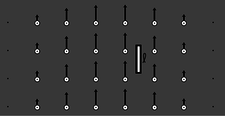p / A classical calculation of the momentum of a light wave. An antenna of length $$\ell$$ is bathed in an electromagnetic wave. The black arrows represent the electric field, the white circles the magnetic field coming out of the page. The wave is traveling to the right.

Note that although some electrons have been set in motion in the wire, we haven't yet seen any momentum transfer, since the protons are experiencing the same amount of electric force in the opposite direction. However, the electromagnetic wave also has a magnetic field, and a magnetic field transfers momentum to (exerts a force on) a current. This is only a force on the electrons, because they're what make the current. The magnitude of this force equals $$\ell IB$$ (homework problem 6), and using the definition of force, $$d\mathbf{p}/dt$$, we find for the magnitude of the momentum transferred:

$\begin{equation*} dp = \ell IB dt \end{equation*}$

We now know both the amount of energy and the amount of momentum that the wave has lost by interacting with the wire. Dividing these two equations, we find

\begin{align*} \frac{dp}{dU} &= \frac{B}{E} \\ &= \frac{1}{c} , \end{align*}

which is what we expected based on relativity. This can now be restated in the form $$d\mathbf{p} = (\text{constant})\mathbf{E}\times\mathbf{B}\,dv$$ (homework problem 40).

Note that although the equations $$p=U/c$$ and $$d\mathbf{p} = (\text{constant})\mathbf{E}\times\mathbf{B}\,dv$$ are consistent with each other for a sine wave, they are not consistent with each other in general. The relativistic argument leading up to $$p=U/c$$ assumed that we were only talking about a single thing traveling in a single direction, whereas no such assumption was made in arguing for the $$\mathbf{E}\times\mathbf{B}$$ form. For instance, if two light beams of equal strength are traveling through one another, going in opposite directions, their total momentum is zero, which is consistent with the $$\mathbf{E}\times\mathbf{B}$$ form, but not with $$U/c$$.q / A simplified drawing of the 1903 experiment by Nichols and Hull that verified the predicted momentum of light waves. Two circular mirrors were hung from a fine quartz fiber, inside an evacuated bell jar. A 150 mW beam of light was shone on one of the mirrors for 6 s, producing a tiny rotation, which was measurable by an optical lever (not shown). The force was within 0.6% of the theoretically predicted value (problem 11 on p. 440) of $$0.001\ \mu\text{N}$$. For comparison, a short clipping of a single human hair weighs $$\sim 1\ \mu\text{N}$$.

Some examples were given in chapter 3 of situations where it actually matters that light has momentum. Figure q shows the first confirmation of this fact in the laboratory.

Angular momentum of light waves

For completeness, we note that since light carries momentum, it must also be possible for it to have angular momentum. If you've studied chemistry, here's an example of why this can be important. You know that electrons in atoms can exist in states labeled s, p, d, f, and so on. What you might not have realized is that these are angular momentum labels. The s state, for example, has zero angular momentum. If light didn't have angular momentum, then, for example, it wouldn't be possible for a hydrogen atom in a p state to change to the lower-energy s state by emitting light. Conservation of angular momentum requires that the light wave carry away all the angular momentum originally possessed by the electron in the p state, since in the s state it has none.

Discussion Questions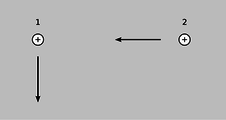Discussion question A.

Positive charges 1 and 2 are moving as shown. What electric and magnetic forces do they exert on each other? What does this imply for conservation of momentum?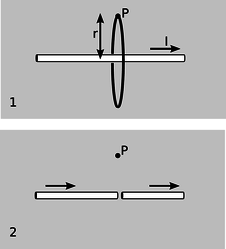Discussion question B.

1. The figure shows a line of charges moving to the right, creating a current $$I$$. An Ampèrian surface in the form of a disk has been superimposed. Use Maxwell's equations to find the field $$B$$ at point P.
2. A tiny gap is chopped out of the line of charge. What happens when this gap is directly underneath the point P?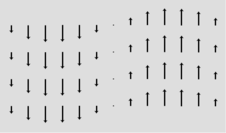Discussion questions C and D

The diagram shows an electric field pattern frozen at one moment in time. Let's imagine that it's the electric part of an electromagnetic wave. Consider four possible directions in which it could be propagating: left, right, up, and down. Determine whether each of these is consistent with Maxwell's equations. If so, infer the direction of the magnetic field.

Discuss what happens to the wave pattern shown in the diagram if we switch to a frame of reference moving along with the wave.

Contributors

Benjamin Crowell (Fullerton College). Conceptual Physics is copyrighted with a CC-BY-SA license.